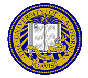# Sample Midterm

These are sample questions that are very similar to the ones I will ask on the midterm.

1. Which of the following is not a valid C variable name?
1. hello
2. chomp_burp
3. whilex
4. more-more-more
5. TrUcKiNg
2. Evaluate the following expressions, assuming a = 1, b = -3, and c = 0. Treat them independently, so (for example) after evaluating (b), use the above values for (c).
1. a + b < c
2. c == !a == c
3. a = ++b; give the values of both a and b as well
4. a-- == !b; give the value of a as well
5. a / b
3. True or False: If x = -1, then if (x) printf("1"); else printf("2"); prints 2.
4. Write a recursive function to add the integers from a to b. Please assume that ab.
5. What are all possible outputs of the following code fragment?
```void f(int a, int b)
{
printf("%d %d\n", a, b);
}

void main(void)
{
int i = 5;
f(i++, i++);
}
```

6. Given the definitions
```    int numbs;
int *ptr = numbs;
```
which of the following are equivalent, and why?
1. numbs
2. numbs + 3
3. *(numbs + 3)
4. *(ptr + 3)
5. *ptr + 3
7. Use the following code fragment to answer parts (a), (b), and (c):
```for(x = i = 0; i <= 100; i += 2, x += i);
```
1. In one short sentence, what does this for loop do?
2. Is the following while loop equivalent? If not, how does its result differ? (Hint: look at the values of both x and i.)
```    x = i = 0;
while( i++ <= 100)
x += ++i;
```
3. Does the following for loop do the same thing? If not, what does it do?
```    for(x = i = 0; i <= 100; i++){
if (!(i % 2))
continue;
x = x + i;
}
```
8. What does this function do?
```char *x(char *s, char c)
{
char *r = NULL;

do{
while(*s && *s != c)
s++;
if (*s)
r = s;
} while(*s++);
return(r);
}
```
9. The following segment of code is supposed to print the number of times the routine a_again is called. Yet, regardless of the input, it prints 0. Why? How would you fix it?
```void a_again(int acount)
{
++acount;
}

int main(void)
{
int c;
int counter = 0;

while((c = getchar()) != EOF)
if (c == 'a' || c == 'A')
a_again(counter);

printf("%d\n", counter);
return(0);
}
```Matt Bishop Office: 2209 Watershed Sciences Phone: +1 (530) 752-8060 Email: mabishop@ucdavis.edu
ECS 36A, Programming and Problem Solving
Version of October 21, 2019 at 6:32PM